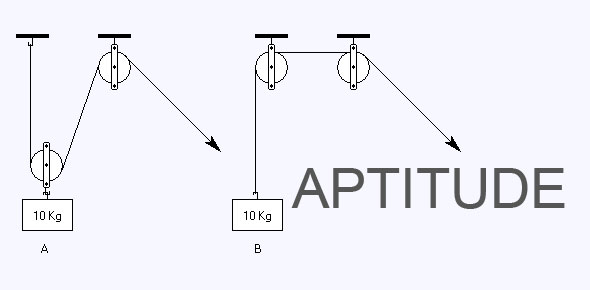# Baja 2016-aptitude-test- 6th Sem

25 Questions | Attempts: 156Settings.

• 1.
A train 125 m long passes a man, running at 5 km/hr in the same direction in which the train is going, in 10 seconds. The speed of the train is:
• A.

45 km/hr

• B.

50 km/hr

• C.

54 km/hr

• D.

55 km/hr

• 2.
Look at this series: 53, 53, 40, 40, 27, 27, ... What number should come next?
• A.

12

• B.

14

• C.

27

• D.

53

• 3.
(1) Tanya is older than Eric. (2) Cliff is older than Tanya. (3) Eric is older than Cliff. If the first two statements are true, the third statement is
• A.

True

• B.

False

• C.

Uncertain

• D.

None

• 4.
A person crosses a 600 m long street in 5 minutes. What is his speed in km per hour?
• A.

3.6

• B.

7.2

• C.

8.4

• D.

10

• 5.
ELFA, GLHA, ILJA, _____, MLNA
• A.

OLPA

• B.

KLMA

• C.

LLMA

• D.

KLLA

• 6.
Here are some words translated from an artificial language. hapllesh means cloudburst srenchoch means pinball resbosrench means ninepin Which word could mean "cloud nine"?
• A.

Leshsrench

• B.

Ochhapl

• C.

Haploch

• D.

Haplresbo

• 7.
In a shower, 5 cm of rain falls. The volume of water that falls on 1.5 hectares of ground is:
• A.

75 cu. m

• B.

750 cu. m

• C.

7500 cu. m

• D.

75000 cu. m

• 8.
Which one of the following is not a prime number?
• A.

31

• B.

61

• C.

71

• D.

91

• 9.
Two numbers are respectively 20% and 50% more than a third number. The ratio of the two numbers is:
• A.

2:5

• B.

3:5

• C.

4:5

• D.

6:7

• 10.
An accurate clock shows 8 o'clock in the morning. Through how may degrees will the hour hand rotate when the clock shows 2 o'clock in the afternoon?
• A.

144º

• B.

150º

• C.

168º

• D.

180º

• 11.
If one-third of one-fourth of a number is 15, then three-tenth of that number is:
• A.

35

• B.

36

• C.

45

• D.

54

• 12.
A man has Rs. 480 in the denominations of one-rupee notes, five-rupee notes and ten-rupee notes. The number of notes of each denomination is equal. What is the total number of notes that he has ?
• A.

45

• B.

60

• C.

75

• D.

90

• 13.
If a - b = 3 and a2 + b2 = 29, find the value of ab.
• A.

10

• B.

12

• C.

15

• D.

18

• 14.
Isometric drawings are often used by ________ to help illustrate complex designs.
• A.

Mechanical engineers

• B.

Piping drafters

• C.

Aerospace engineers

• D.

All of the above

• 15.
The average weight of A, B and C is 45 kg. If the average weight of A and B be 40 kg and that of B and C be 43 kg, then the weight of B is:
• A.

17 kg

• B.

20 kg

• C.

26 kg

• D.

31 kg

• 16.
What smallest number should be added to 4456 so that the sum is completely divisible by 6 ?
• A.

4

• B.

3

• C.

2

• D.

1

• 17.
The sum of two number is 25 and their difference is 13. Find their product.
• A.

104

• B.

114

• C.

315

• D.

325

• 18.
397 x 397 + 104 x 104 + 2 x 397 x 104 = ?
• A.

250001

• B.

251001

• C.

260101

• D.

261001

• 19.
(xn - an) is completely divisible by (x - a), when
• A.

N is any natural number

• B.

N is an even natural number

• C.

N is and odd natural number

• D.

N is prime

• 20.
In the first 10 overs of a cricket game, the run rate was only 3.2. What should be the run rate in the remaining 40 overs to reach the target of 282 runs?
• A.

6.25

• B.

6.5

• C.

6.75

• D.

7

• 21.
A person crosses a 600 m long street in 5 minutes. What is his speed in km per hour?
• A.

3.6

• B.

7.2

• C.

8.4

• D.

10

• 22.
'Dandia' is a popular dance of
• A.

Punjab

• B.

Gujarat

• C.

• D.

Maharashtra

• 23.
(1) A fruit basket contains more apples than lemons. (2) There are more lemons in the basket than there are oranges. (3) The basket contains more apples than oranges. If the first two statements are true, the third statement is
• A.

True

• B.

False

• C.

Uncertain

• D.

None

• 24.
B2CD, _____, BCD4, B5CD, BC6D
• A.

B2C2D

• B.

BC3D

• C.

B2C3D

• D.

BCD7

• 25.
Logical Reasoning Question - Which word does NOT belong with the others?
• A.

Inch

• B.

Pound

• C.

Centimeter

• D.

Yard

## Related TopicsBack to top
×

Wait!
Here's an interesting quiz for you.